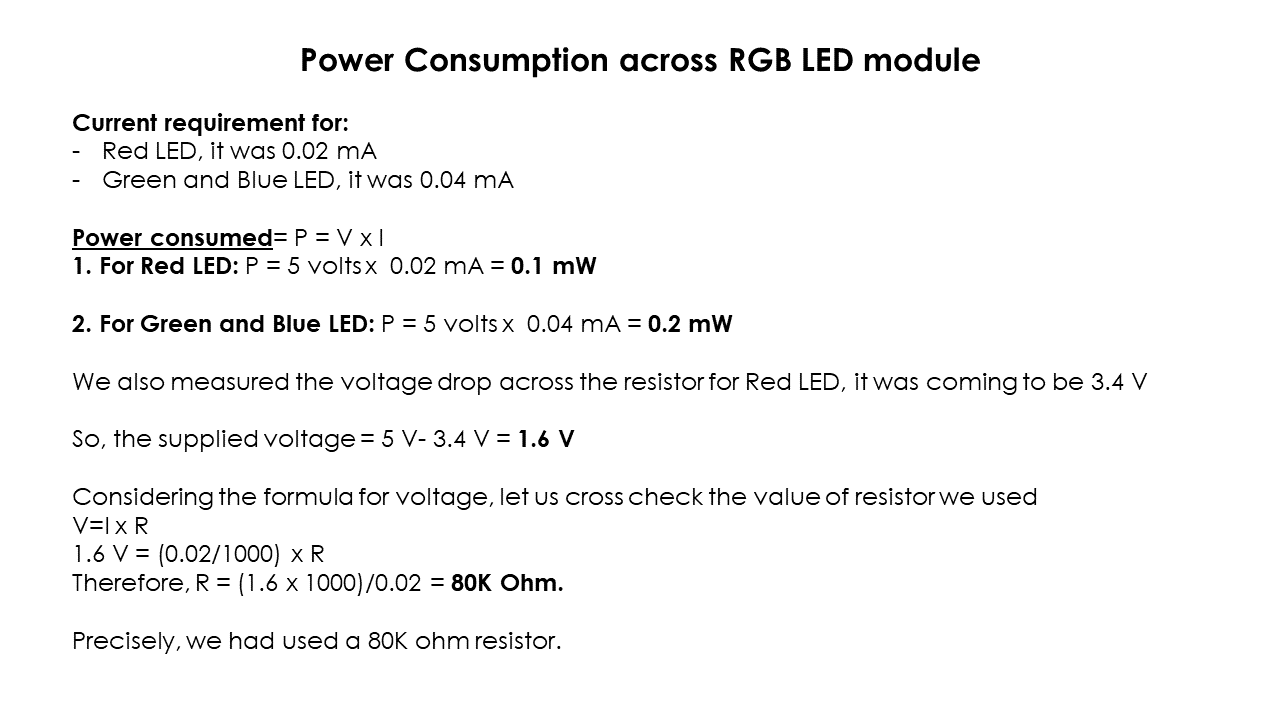### Group Assignment #8: Output Devices

This assignment is about documenting what we learned in Output Devices week that includes understanding different output devices and measure the power consumption of an output device on one of the board we made as a part of individual assignment for this week.

##### Objectives of the Group Assignment:

- Measure the power consumption of an output device

##### Output Devices that we measured the power consumption of:

- DC motor
- RGB LED

1. We connected DC motor to the output devices board and ran the motor after programming the board through arduino IDE. First we measured current in series and then we measured current in series and voltage across parallel connection as shown below.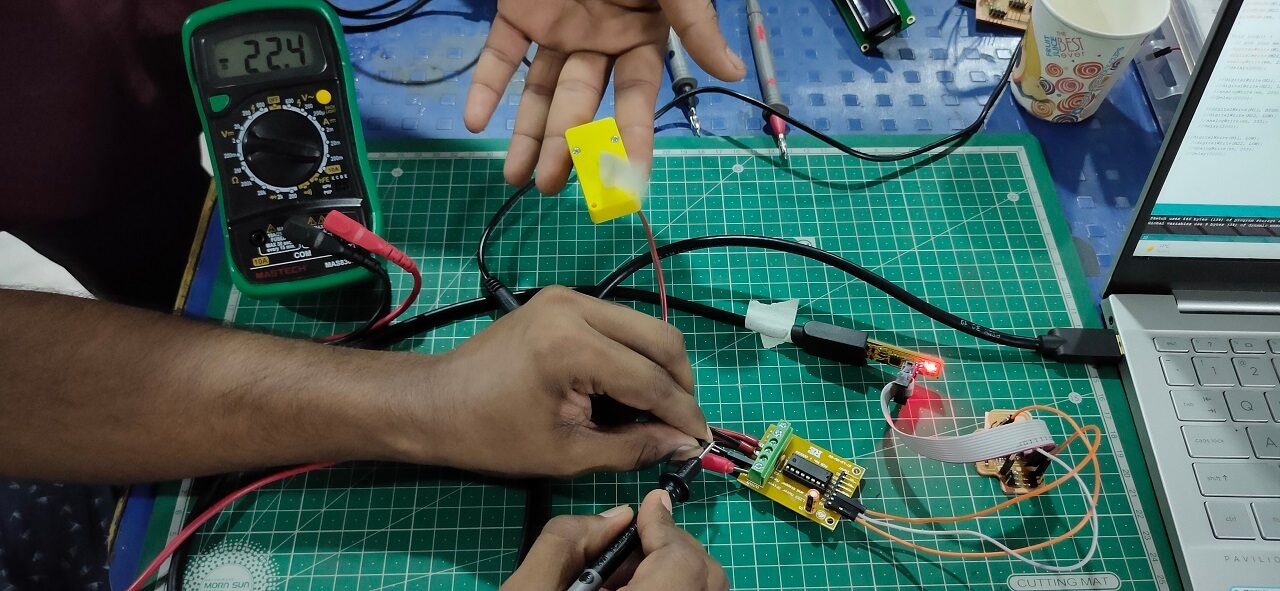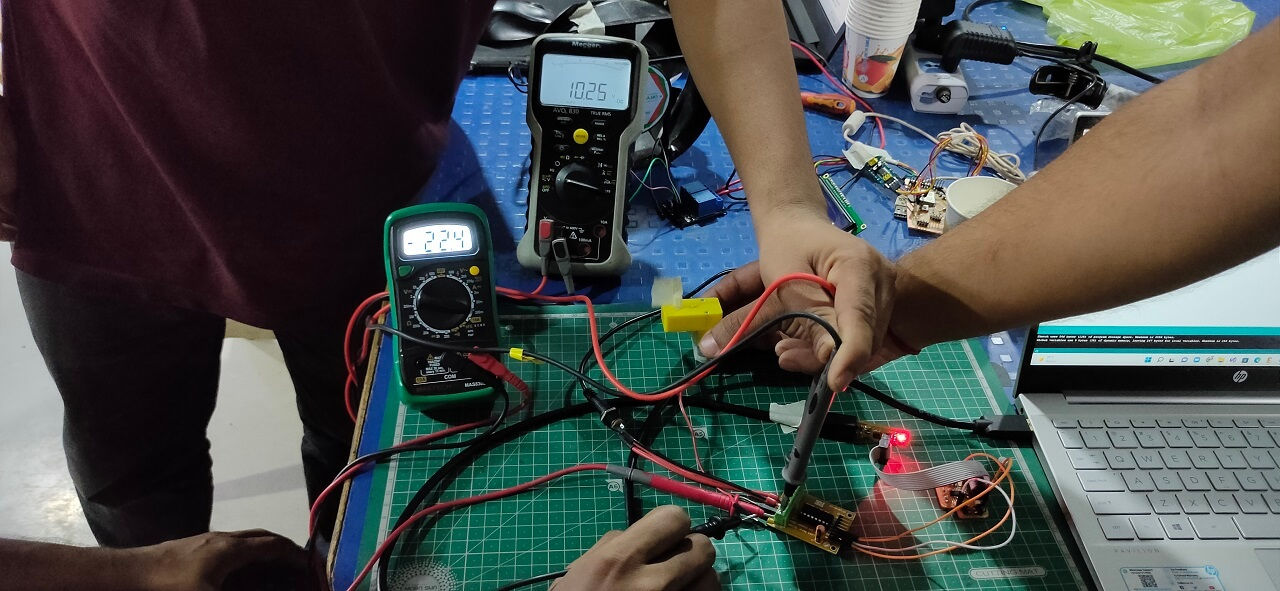Power consumption of a DC motor: The measured voltage (V) for the DC motor was 10.25 Volts and the value of current required (I) was varying. However, the one, which was most consistent was 22.4 mA. So the power consumption of a DC toy motor= P= V x I = 10.25 x 0.0224 A = 0.2296 Watts.

2. In second case, we connected RGB LED module to the output devices board and blinked each LED (Red, Green and Blue) separately after programming the board through arduino IDE. Also measured the current by attaching the resistor of 80K in series with the help of Megger make multimeter as shown below.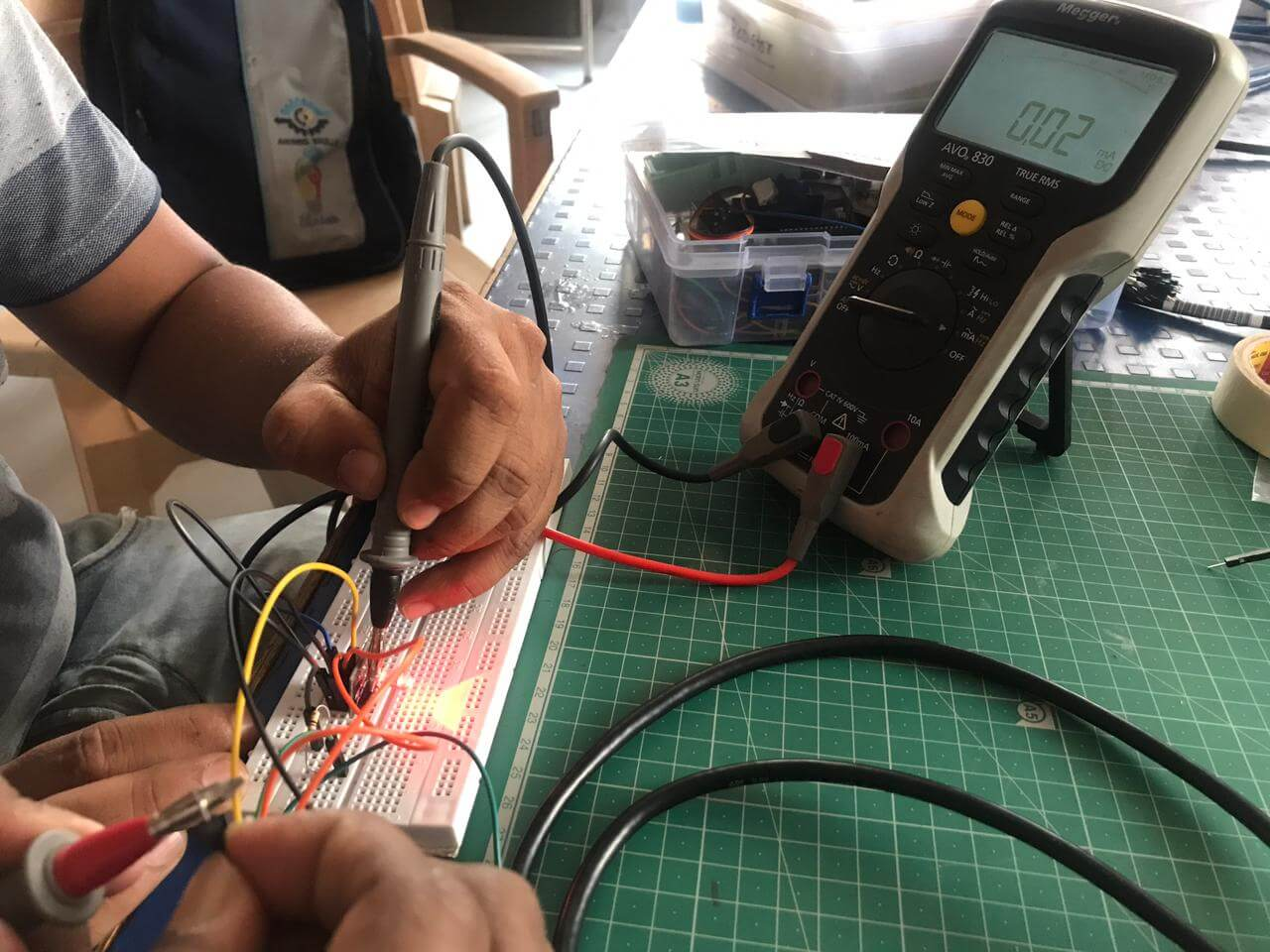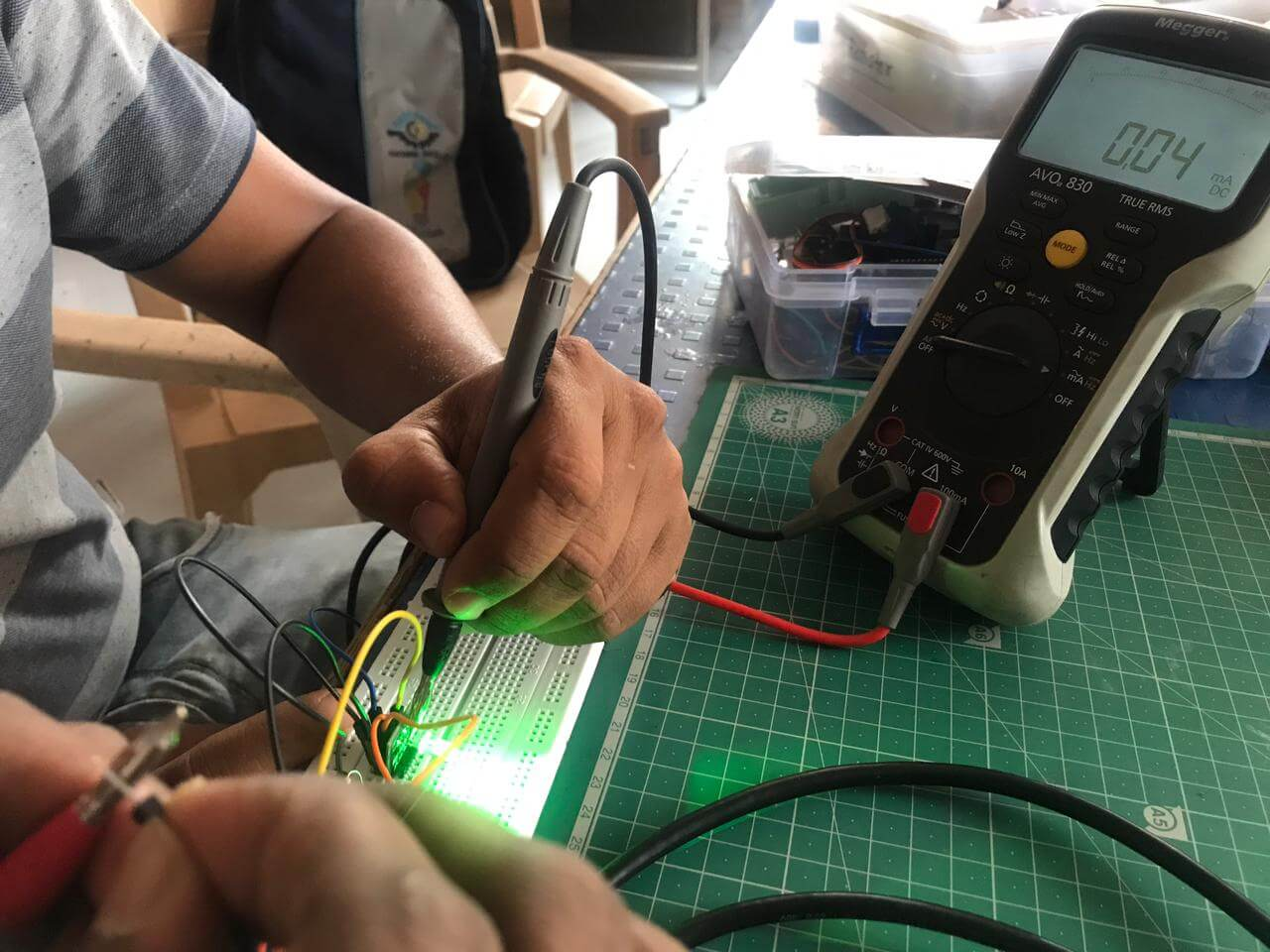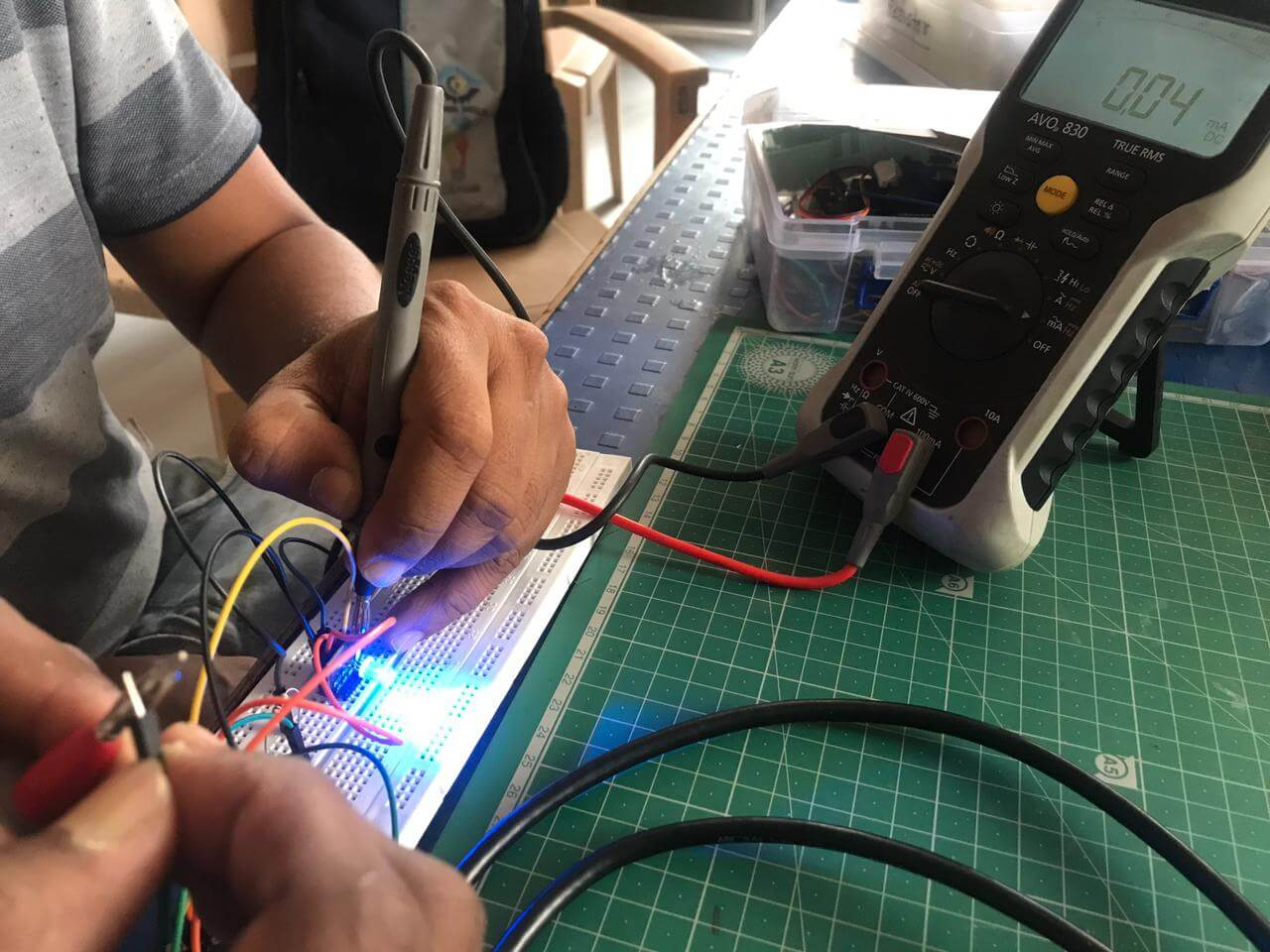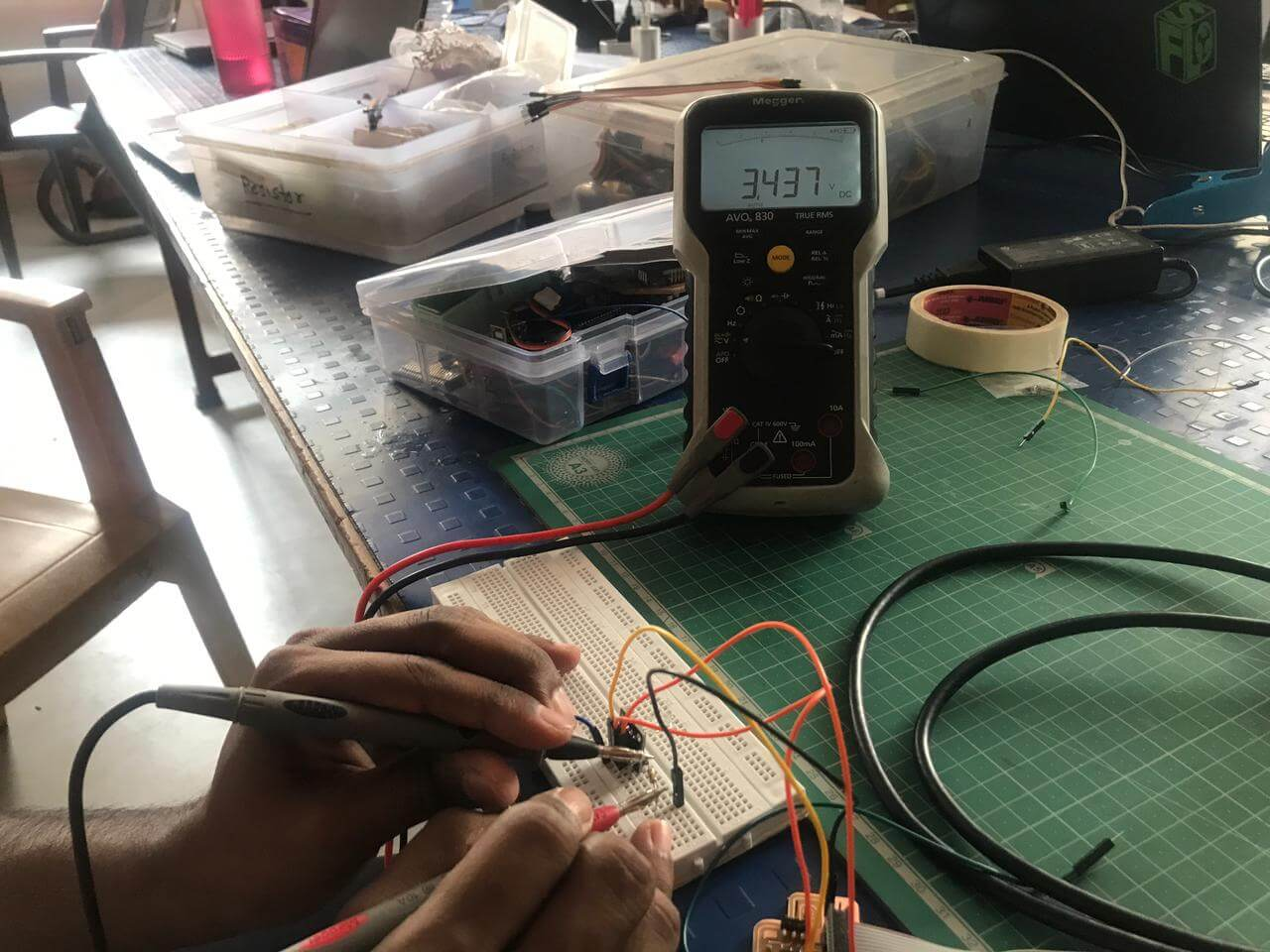Power consumption of RGB LED: The operating voltage (V) for RGB LED was 5 Volts and the value of current (I) was different for Red, Green and Blue LED. For Red LED, it was 0.02 mA, for Green and Blue LED, it was 0.04 mA. Following are our calculations of power consumption of RGB LED.